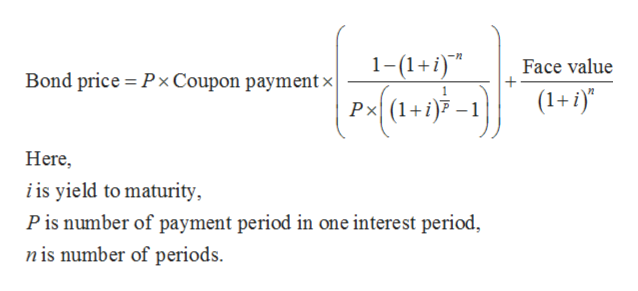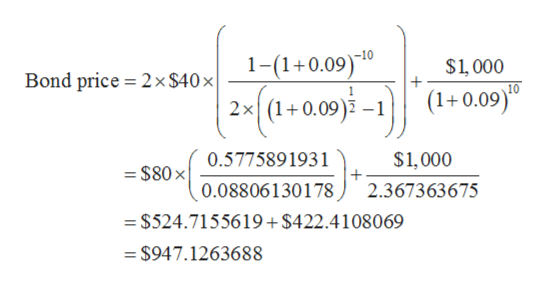# A bond has an annual 8 percent coupon rate, a maturity of 10 years, a face value of \$1,000, and makes semiannual payments.   If the price is \$934.96, what is the annual nominal yield to maturity on the bond?

Question
9 views

A bond has an annual 8 percent coupon rate, a maturity of 10 years, a face value of \$1,000, and makes semiannual payments.   If the price is \$934.96, what is the annual nominal yield to maturity on the bond?

check_circle

Step 1

The formula to calculate price of bond is given below,help_outlineImage Transcriptionclose1-(1+) Face value Bond price Px Coupon paymentx Px(1+aP-1 (1+i) Here i is yield to maturity Pis number of payment period in one interest period nis number of periods fullscreen
Step 2

Substitute \$40 for coupon payment, 2 for P, 10 for n and \$1,000 for face value,

Step 3

Now calculate bond price a...help_outlineImage Transcriptionclose1-(1+0.09)10 \$1,000 + 2x \$40 x| Bond price (1+0.09)0 2x (1+0.09 1 \$1,000 0.5775891931 = \$80x 0.08806130178 2.367363675 \$524.7155619+\$422.4108069 =\$947.1263688 fullscreen

### Want to see the full answer?

See Solution

#### Want to see this answer and more?

Solutions are written by subject experts who are available 24/7. Questions are typically answered within 1 hour.*

See Solution
*Response times may vary by subject and question.
Tagged in